## 零重力条件下低温射流抑制大尺寸液氢储罐热分层的数值研究1)

*中国科学院大学工程科学学院,北京 100049

**中国科学院力学研究所高温气体动力学国家重点实验室,北京 100190

## NUMERICAL STUDY ON THERMAL DESTRATIFICATION IN LARGE SCALE HYDROGEN PROPELLANT TANK IN SPACE BY JET INJECTION UNDER ZERO GRAVITY CONDITION1)

Guo Bin,*,,2), Zhao Jianfu*,,**, Li Kai,*,,**,3), Hu Wenrui*,

*School of Engineering Science,University of Chinese Academy of Sciences,Beijing 100049,China

National Laboratory of Microgravity,Institute of Mechanics,CAS, Beijing 100190,China

**State Key Laboratory of High Temperature Gas Dynamics,Institute of Mechanics,CAS,Beijing 100190,China

 基金资助: 1)国家自然科学基金.  11672311中国科学院前沿科学重点研究.  QYZDY-SSWJSC040

Received: 2020-09-29   Accepted: 2021-01-7   Online: 2021-04-08Abstract

Liquid Hydrogen plays a vital role in the future energy system as a space propellant, but it is sensitive to heat leakage from the environment because of its low boiling point and easy evaporation. On the other hand the buoyancy convection in the space microgravity environment is significantly reduced. When there is local heat leakage on the wall of the propellant tank, temperature stratification arises around the heat leakage source causing local overheating. It seriously affects the multiphase heat and mass transfer in the propellant tank which induces the tank pressure rise and jeopardize the structural safety of the system. To prevent the tank pressure from rising above the design of limits, venting or active pressure control techniques must be implemented. The cryogenic jet mixing is an effective means to suppress temperature stratification. The cryogenic fluid is mixed with the fluid inside the tank through a jet nozzle to reduce the local high temperature and achieve uniform temperature. In present paper, the thermal stratification phenomenon caused by the local heat leakage under microgravity condition was numerically simulated by using a fully filled two-dimensional large scale tank model. This paper mainly analyzes the suppression and elimination of local thermal stratification caused by heat leakage near the bottom of the storage tank and the outlet connecting section. The influence of different cryogenic jet mixing conditions on eliminating the temperature stratification effect is analyzed. The results show that the position of jet nozzle has no obvious effect on the elimination of thermal stratification inside the tank when the circular jet nozzle is used and the cryogenic jet condition is the same. When the jet nozzles are located in the same relative position inside the tank and the incident flow rate is the same, the circular jet nozzles have more concentrated flow direction, the flow field in the tank evolves faster, so the thermal stratification elimination effect is better than the hemispherical jet nozzles.

Keywords： zero gravity ; cryogenic propellant ; thermal destratification ; cryogenic jet mixing

Guo Bin, Zhao Jianfu, Li Kai, Hu Wenrui. NUMERICAL STUDY ON THERMAL DESTRATIFICATION IN LARGE SCALE HYDROGEN PROPELLANT TANK IN SPACE BY JET INJECTION UNDER ZERO GRAVITY CONDITION1). Chinese Journal of Theoretical and Applied Mechanics[J], 2021, 53(4): 1170-1182 DOI:10.6052/0459-1879-20-343

## 1 计算模型

### 图1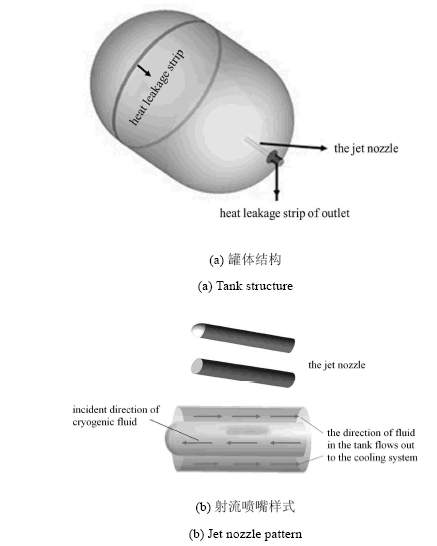Fig.1   Schematic diagram of tank structure and jet nozzle pattern

### 图2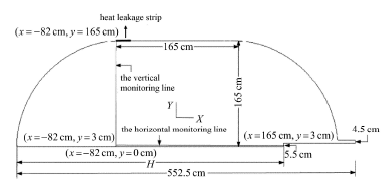Fig.2   Schematic diagram of the cryogenic storage tank and inspection line

### 1.2 数学模型

1.2.1 控制方程

(1) 连续方程

$\dfrac{1}{r}\dfrac{\partial }{\partial r}(ru_{ r}) + \dfrac{\partial u_{ z} }{\partial z} = 0$

(2) 动量方程

$\rho \left(\dfrac{\partial u_{ r} }{\partial t} + u_{ r} \dfrac{\partial u_{ r} }{\partial r} + u_{ z} \dfrac{\partial u_{ r} }{\partial z}\right) = \dfrac{1}{r}\dfrac{\partial }{\partial r}(r\sigma _{ rr} ) - \dfrac{\sigma _{\theta \theta } }{r} + \dfrac{\partial \sigma _{ rz} }{\partial z}$
$\rho \left(\dfrac{\partial u_{ z} }{\partial t} + u_r \dfrac{\partial u_{ z} }{\partial r} + u_{ z} \dfrac{\partial u_{ z} }{\partial z}\right) = \dfrac{1}{r}\dfrac{\partial }{\partial r}(r\sigma _{ rz} ) + \dfrac{\partial \sigma _{ zz} }{\partial z}$

$\sigma _{ rr} = - p + 2(\mu + \mu _{t} )\dfrac{\partial u_r }{\partial r}$
$\sigma _{\theta \theta } = - p + 2(\mu + \mu _{t} )\dfrac{u_r }{r}$
$\sigma _{ rz} = (\mu + \mu _{t})\left(\dfrac{\partial u_r }{\partial z} + \dfrac{\partial u_z }{\partial r}\right )$
$\sigma _{ zz} = - p + 2(\mu + \mu _{t})\dfrac{\partial u_z }{\partial z}$

$\mu _{t} = \rho C_\mu \dfrac{k^2}{\varepsilon }$

(3) 能量方程

$\rho c_{p} \left (\dfrac{\partial T}{\partial t} + u_{r} \dfrac{\partial T}{\partial r} + u_{z} \dfrac{\partial T}{\partial z}\right ) = \dfrac{1}{r} \dfrac{\partial }{\partial r} \left[ {(k + k_{t} )r\dfrac{\partial T}{\partial r}} \right] + \dfrac{\partial }{\partial z}\left[ {(k + k_{t} )\dfrac{\partial T}{\partial z}} \right]$
$k_{t} = \dfrac{c_{p} \mu _{t} }{pr_{t} }$

1.2.2 湍流模型

$\dfrac{\partial }{\partial t}(\rho k) + \nabla \cdot (\rho k) = \nabla \cdot \left[ {\left (\mu + \dfrac{\mu _{t} }{\sigma _{k} }\right )\nabla k} \right] + G_{k} - \rho \varepsilon - D_{k}$
$\dfrac{\partial }{\partial t}(\rho \varepsilon ) + \nabla \cdot (\rho \varepsilon ) = \nabla \cdot \left[ {\left (\mu + \dfrac{f_\mu \mu _{t} }{\sigma _\varepsilon }\right )\nabla \varepsilon } \right] + C_1 \dfrac{\varepsilon }{k} G_{k} - f_2 C_2 \rho \dfrac{\varepsilon ^2}{k}$

$G_{k} = f_\mu \mu _{t} \nabla \bar v ( \overline{\nabla v} + \nabla \bar v^{T})$

$D_{k}$表征湍动能在黏性层中各项异性衰减的影响, 在AB模型中

$D_{k} = 2\mu \left(\dfrac{\partial k^{1 / 2}}{\partial r}\right )$

$f_\mu = \exp ( - \dfrac{2.5}{1 + Re_{t}/ 50})$
$f_2 = 1.0 - 0.3\exp ( - Re_{t}^2)$
$C_1 = 1.44 , \ \ C_2 = 1.92 , \ \ \sigma _{k} = 1.0 , \ \ \sigma _\varepsilon = 1.3$

$Re_{t} = \dfrac{\rho k^2}{\varepsilon \mu }$

1.2.3 边界条件以及初始条件

$u_{ r} = 0 , \ \ u_{ z} = U_{in} , \ \ T_{in} = 16K$

$u_{r} = 0 , \ \ \dfrac{\partial u_{z} }{\partial r} = 0 , \ \ \dfrac{\partial T}{\partial r} = 0$

$u_{r} = u_{z} = 0 , \ \ T= 25 {K}$

$u_{r} = u_{z}= 0 , \ \ \dfrac{\partial T}{\partial n} = 0$

$T_0 = 18 {K}$

Table 1  Thermal physical property values of liquid hydrogen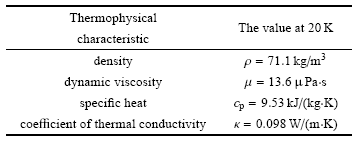## 2 数值模拟

### 图3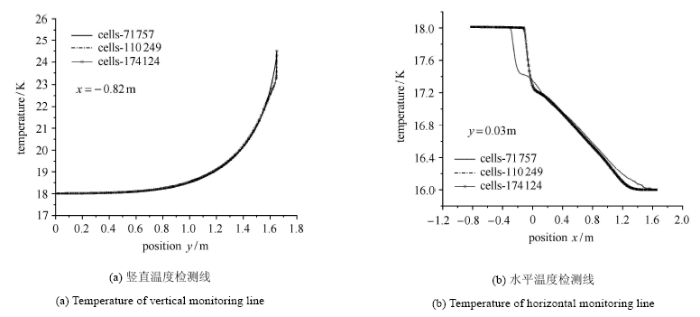Fig.3   Grid independence test

## 3 结果讨论

Table 2  Details of computational cases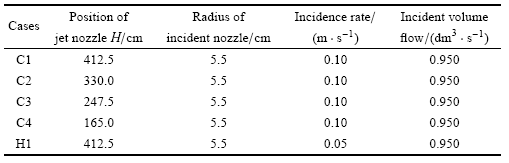### 图4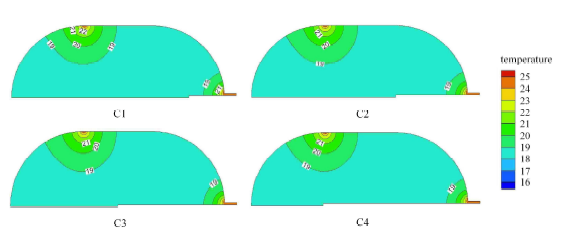Fig.4   Isothermals of the initial state ($t=0$ s) for the cryogenic jet

### 图5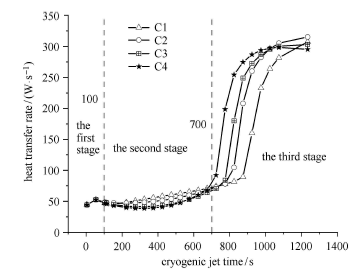Fig.5   Evolution diagram of the heat transfer rate with cryogenic jet time in the circular jet nozzle

### 图6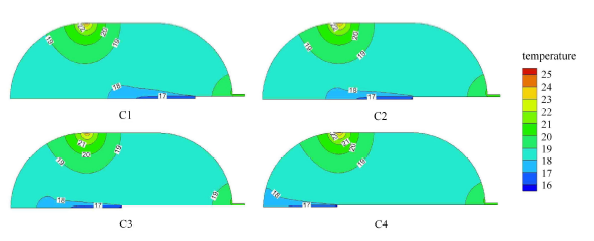Fig.6   Isothermals of cases at $t=64.08$ s for the cryogenic jet

### 图7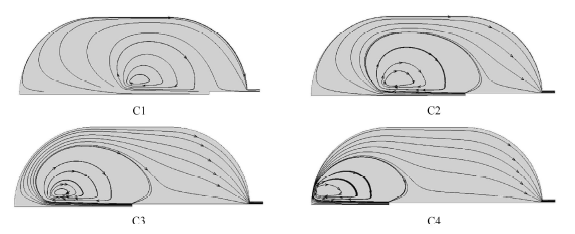Fig.7   Flow field diagram at $t=64.08$ s for the cryogenic jet

### 图8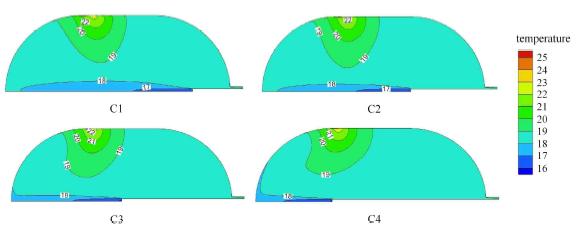Fig.8   Isothermals of cases at $t=424.08$ s for the cryogenic jet

### 图9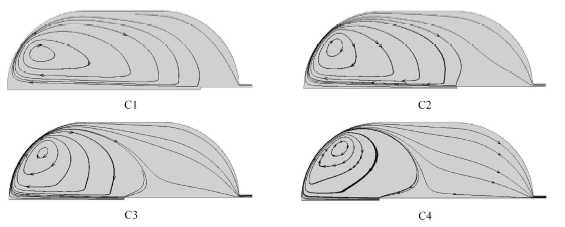Fig.9   Flow field diagram at $t=424.08$ s for the cryogenic jet

### 图10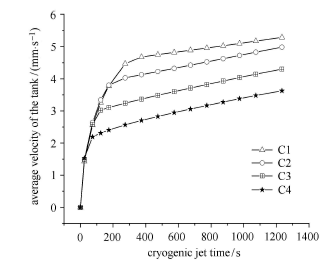Fig.10   Evolution diagram of the average velocity of the tank with the cryogenic jet time in the circular jet nozzle

### 图11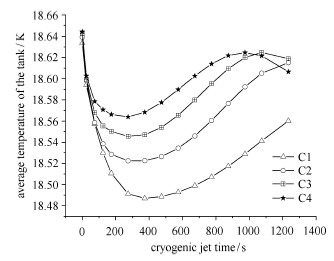Fig.11   Evolution diagram of the average temperature of the tank with the cryogenic jet time in the circular jet nozzle

### 图12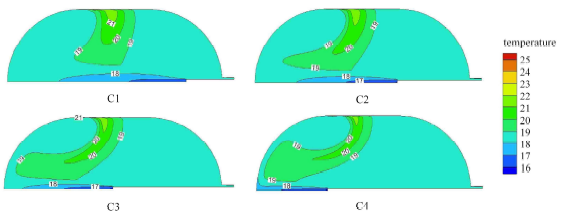Fig.12   Isothermals of cases at $t=1 024.08$ s for the cryogenic jet

### 图13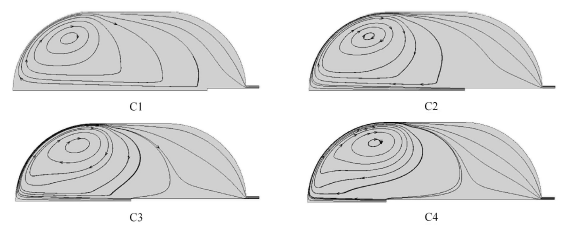Fig.13   Flow field diagram at $t=1 024.08$ s for the cryogenic jet

### 图14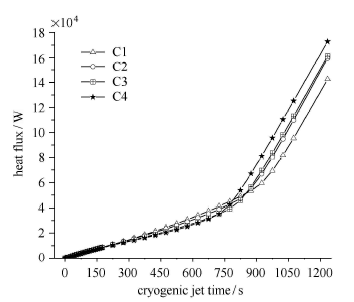Fig.14   Evolution diagram of heat flux over time for each circular nozzle case

### 图15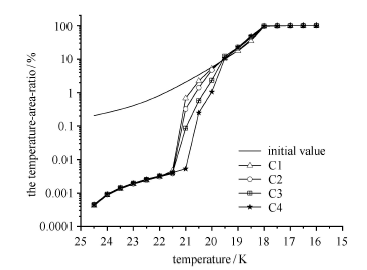Fig.15   Comparison of the cumulative curve of the temperature-area-ratio for each circular nozzle case

### 图16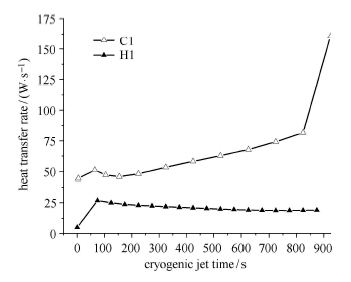Fig.16   Contrast diagram of temperature stratification of circular and hemispherical jet nozzles

### 图17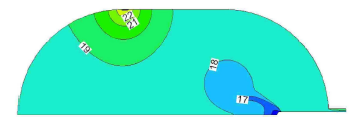Fig.17   Isothermals of H1 at $t=424.08$ s for the cryogenic jet

### 图18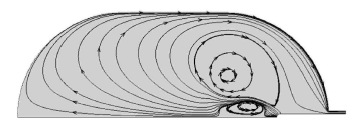Fig.18   Flow field diagram of H1 at $t=424.08$ s for the cryogenic jet

## 参考文献 原文顺序 文献年度倒序 文中引用次数倒序 被引期刊影响因子

Hastings LJ, Plachta DW, Salerno L, et al.

An overview of NASA efforts on zero boiloff storage of cryogenic propellants

Cryogenics, 2001,41(11-12):833-839

Poth LJ, Hook JRV.

Control of the thermodynamic state of space-stored cryogens by jet mixing

Journal of Spacecraft and Rockets, 1972,9(5):332-336

Merte H, Clark JA, Barakat HZ.

Finite difference solution of stratification and pressure rise in containers. Tech. rep

Heat Transfer Laboratory, University of Michigan, 1968

Audelott JC. Axial jet mixing of ethanol in spherical containers during weightlessness. NASA TP-1487, 1979

Lin CS, Hasan MM, Vandresar NT. Experimental investigation of jet-induced mixing of a large liquid hydrogen storage tank. Nasa Sti/recon Technical Report N, 1994,94

Panzarella C, Kassemi M. Simulations of zero boil-off in a cryogenic storage tank//41st Aerospace Sciences Meeting and Exhibit, 2003

Rahman M, Mukka S. Computation of fluid circulation in a cryogenic storage vessel//2nd International Energy Conversion Engineering Conference, 2004

Ho SH, Rahman MM.

Three-dimensional analysis for liquid hydrogen in a cryogenic storage tank with heat pipe-pump system

Cryogenics, 2008,48(1-2):31-41

Ho SH, Rahman MM.

Nozzle injection displacement mixing in a zero boil-off hydrogen storage tank

International Journal of Hydrogen Energy, 2008,33(2):878-888

Ho SH, Rahman MM.

Forced convective mixing in a zero boil-off cryogenic storage tank

International Journal of Hydrogen Energy, 2012,37(13)

Belmedani M, Belgacem A, Rebiai R.

Analysis of natural convection in liquid nitrogen under storage conditions

Journa of Applied Science, 2008,8(14):2544-2552

Zilliac G, Karabeyoglu M. Modeling of propellant tank pressurization//AIAA/ASME/SAE/ASEE Joint Propulsion Conference & Exhibit, 2013

Grayson G, Lopez A, Chandler F, et al. CFD modeling of helium pressurant effects on cryogenic tank pressure rise rates in normal gravity//43rd AIAA/ASME/SAE/ASEE Joint Propulsion Conference & Exhibit, 2007

Barsi S, Panzarella C, Kassemi M. An active vapor approach to modeling pressurization in cryogenic storage tanks//AIAA/ ASME/SAE/ASEE Joint Propulsion Conference & Exhibit, 2007

Lopez A, Grayson G, Chandler F, et al. Cryogenic pressure control modeling for ellipsoidal space tanks//43rd AIAA/ASME/ SAE/ASEE Joint Propulsion Conference & Exhibit, 2007

Barsi S, Kassemi M.

Numerical and experimental comparisons of the self-pressurization behavior of an LH2 tank in normal gravity

Cryogenics, 2008,48(3-4):122-129

Kumar SP, Prasad BVSSS, Venkatarathnam G, et al.

Influence of surface evaporation on stratification in liquid hydrogen tanks of different aspect ratios

International Journal of Hydrogen Energy, 2007,32(12):1954-1960

Oliveira JM, Kirk DR, Schallhorn P.

Analytical model for cryogenic stratification in a rotating and reduced-gravity environment

Journal of Spacecraft and Rockets, 2009,46(2):459-465

Li X, Xie G, Wang R.

Experimental and numerical investigations of fluid flow and heat transfer in a cryogenic tank at loss of vacuum

Heat and Mass Transfer, 2010,46(4):395-404

Wang XJ, Yuan XZ, Xu SH, et al.

Numerical study of zero boil-off storage system with heat pipe and pump-nozzle unit

HVAC&R Research, 2014,20(3):320-327

Liu YW, Liu X, Yuan XZ, et al.

Optimizing design of a new zero boil off cryogenic storage tank in microgravity

Applied Energy, 2016,162:1678-1686

Liu YW, Wu RJ, Yang P, et al.

Parameter study of the injection configuration in a zero boil-off hydrogen storage tank using orthogonal test design

Applied Thermal Engineering, 2016,109:283-294

Roh S, Son G, Song G, et al.

Numerical study of transient natural convection in a pressurized LNG storage tank

Applied Thermal Engineering, 2013,52(1):209-220

Wang L, Li Y, Li C, et al.

CFD investigation of thermal and pressurization performance in LH2 tank during discharge

Cryogenics, 2013,57(Complete):63-73

Wang L, Li Y, Zhao Z, et al.

Transient thermal and pressurization performance of LO2 tank during helium pressurization combined with outside aerodynamic heating

International Journal of Heat and Mass Transfer, 2013,62:263-271

Daigle MJ, Smelyanskiy VN, Boschee J, et al.

Temperature stratification in a cryogenic fuel tank

Journal of Thermophysics and Heat Transfer, 2013,27(1):116-126

Fu J, Sunden B, Chen X.

Influence of wall ribs on the thermal stratification and self-pressurization in a cryogenic liquid tank

Applied Thermal Engineering, 2014,73(2):1421-1431

Fu J, Sunden B, Chen X, et al.

Influence of phase change on self-pressurization in cryogenic tanks under microgravity

Applied Thermal Engineering, 2015,87:225-233

(Li Jiachao, Liang Guozhu.

Experiment and numerical simulation of liquid nitrogen tank self pressurization

Journal of Astronautics, 2018,29(4):426-434 (in Chinese))

(Wang Xi, Wang Jue, Rong Yi, et al.

Computational research on phase change model for cryogenic propellant in microgravity

Missiles and Space Vehicles, 2018(1):38-40 (in Chinese))

(Li Peng, Sun Peijie, Sheng Minjian, et al.

Investigation on passive boil-off control scheme for orbital storage of cryogenic propellant in orbital transfer spacecraft

Manned Spaceflight, 2018,24(1):91-97 (in Chinese))

(Guo Zhifan, Ju Yonglin.

Status and problems of cryogenic liquid hydrogen storage

Cryogenics & Superconductivity, 2019(6):21-29 (in Chinese))

(Ma Yuan, Sun Peijie, Li Peng, et al.

Numerical investigation on performance of spraying pressure control technique for liquid hydrogen tank at microgravity

Vacuum and Cryogenics, 2018,24(4):266-274 (in Chinese))

(Wang Shunhao, Zhu Wenli, Hu Zhenggen, et al.

Numerical simulation and experimental validation of evaporation characteristics of scaled liquid hydrogen tank

CIESC Journal, 2018,70(3):840-849 (in Chinese))

Zuo ZQ, Sun PJ, Jiang WB, et al.

Thermal stratification suppression in reduced or zero boil-off hydrogen tank by self-spinning spray bar

International Journal of Hydrogen Energy, 2019,44(36)

(Guo Bin, Zhao Jianfu, Li Kai, et al.

Thermal destratification in hydrogen propellant tank in space by jet injection

Chinese Journal of Space Science, 2020,40(6):1052-1065 (in Chinese))

(Zhang Manman, Sun Jiao, Chen Wenyi.

An interface tracking method of coupled youngs-vof and level set based on geometric reconstruction

Chinese Journal of Theoretical and Applied Mechanics, 2019,51(3):775-786 (in Chinese))

Abid R.

Assessment of two-equation turbulence models for predicting transitional flows

International Journal of Engineering Science, 1993,31(6):831-840

/

 〈〉